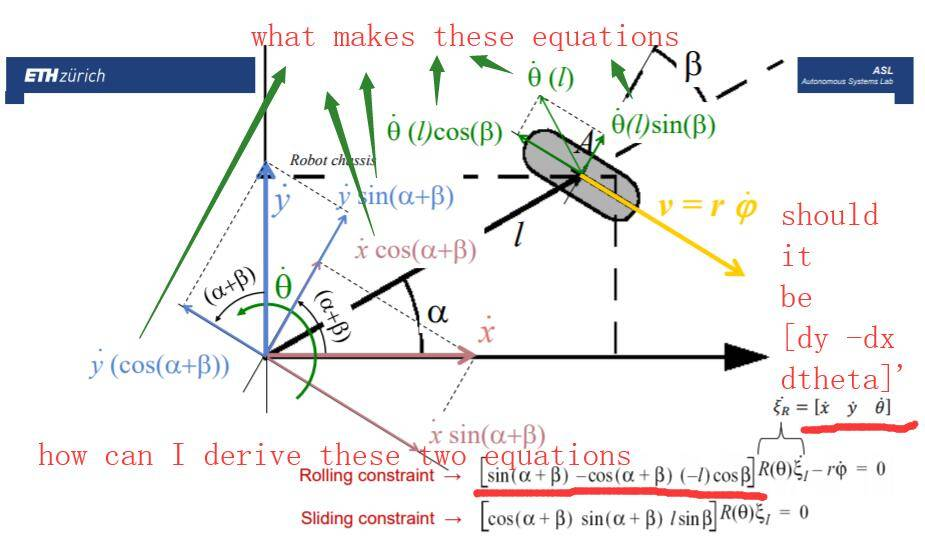# Problems in understanding kinematics

arifle
TL;DR Summary
wheeled robot kinematics and constraints
Hi, I tried to understand kinematics after having an omnidirectional roobt. Some problems stop me to go further. Here I upload some contents of different papers talking about kinematics. For the 1st three pictures, I don't know how equation (8) is from and I am little confused about translational and tangentianal velocities. I don't know why it mutiplys R(theta) again in equation (8).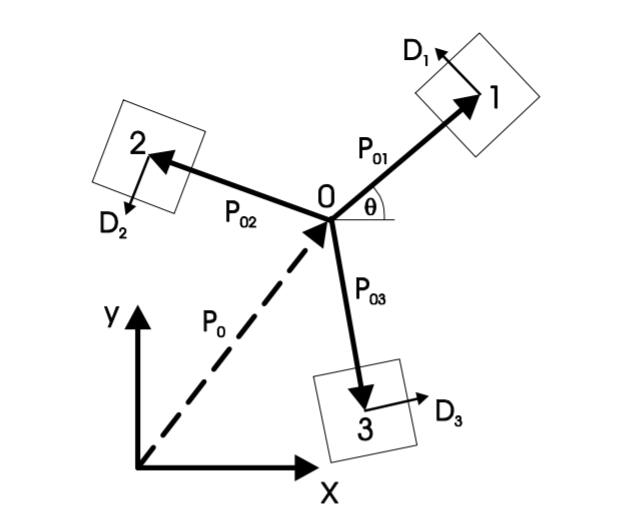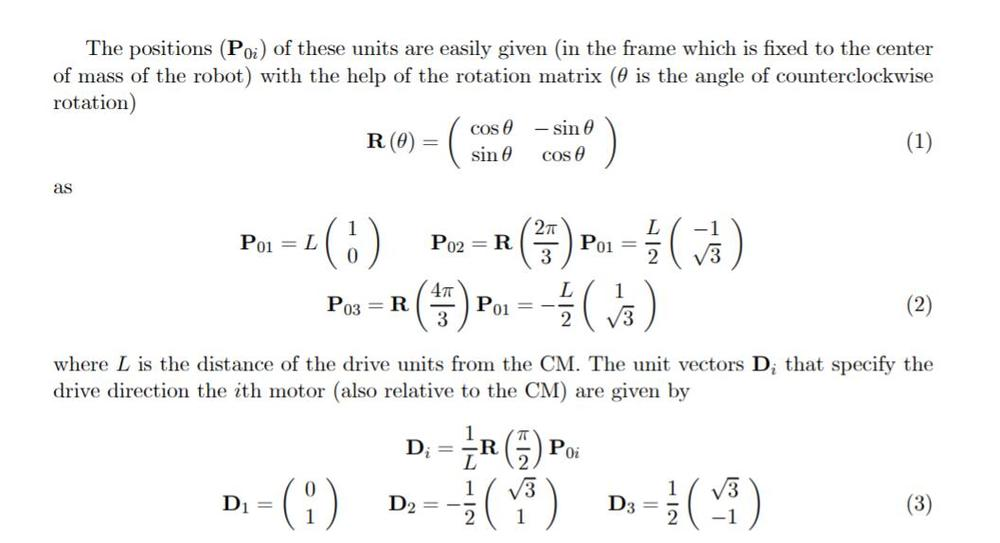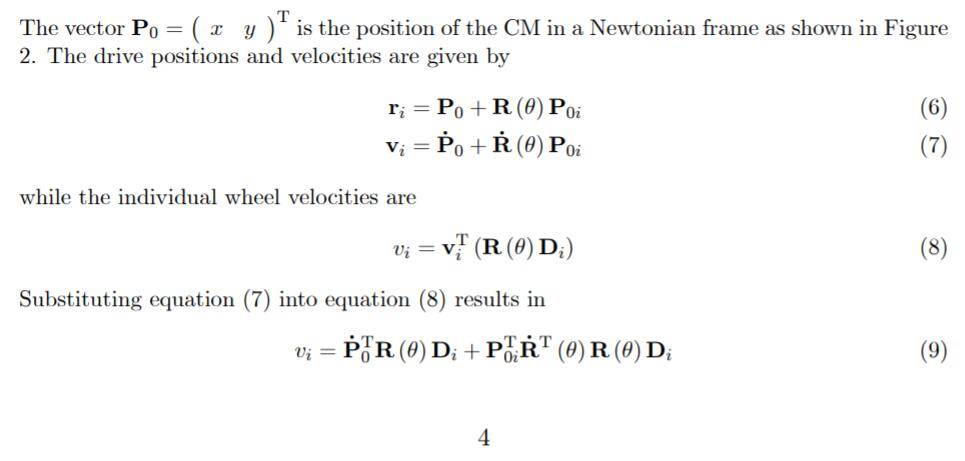For the 2nd paper, I cannot obtain the equation of v_trans,i. Also, I am not sure if the rotation matrix in this case is R(theta) = [cos(theta) -sin(theta); sin(theta) cos(theta)]. Can anyone please tell some details about the kinematics.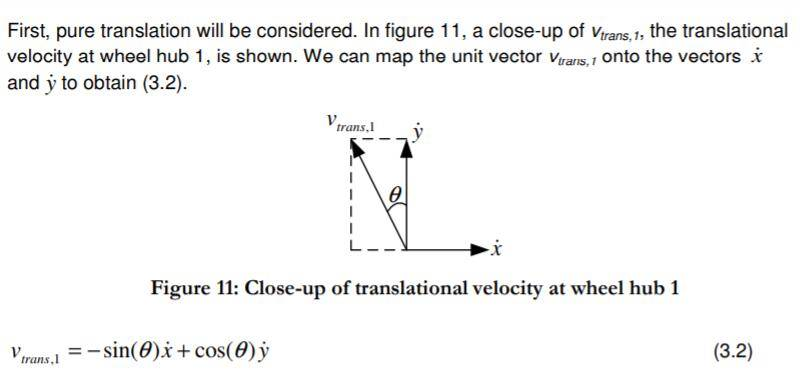At last, there is another lecture about kinematics. The problem is still about the constraints. How can I derive this pure rolling constraint.